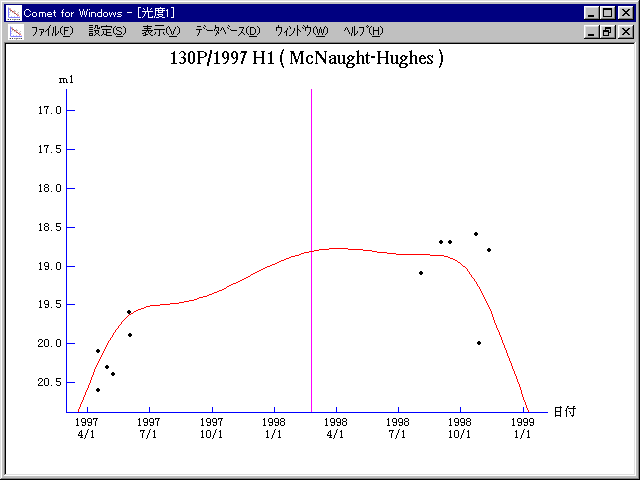# \$B%^%/%N!<%H(B-\$B%R%e!<%:WB@1(B

130P/McNaught-Hughes (1998)

 English version Home page Updated on March 26, 1999###\$B%W%m%U%#!<%k(B

 \$BId9f(B 130P/1997 H1 \$B8!=PF|(B 1997\$BG/(B4\$B7n(B16\$BF|(B, 4\$B7n(B29\$BF|(B \$B8!=P8wEY(B 20.6\$BEy(B, 20.3\$BEy(B \$B8!=P J. V. Scotti, J. Larsen (Kitt Peak), \$BCfB<>4@5(B(\$B5WK|9b86(B)

###\$B50F;MWAG(B

```   The following improved orbital elements by Kenji Muraoka, are
from 91 observations 1991 Sept. 14 to 1999 Jan. 15, perturbations
by 9 Plantes, Moon and 5 minor planets were taken into account.
The mean residual is +/- 0.57 arc seconds.

Epoch  =  1998 Mar.  8.0  TT       JDT = 2450880.5
T  =  1998 Feb. 23.78371       +/- 0.00025 (m.e.) TT
Peri. =  224.36816                +/- 0.00022
Node  =   89.97248                +/- 0.00022   (2000.0)
Incl. =    7.30336                +/- 0.00002
q  =    2.1162919              +/- 0.0000004 AU
e  =    0.4041126              +/- 0.0000001
a  =    3.5514967              +/- 0.0000003 AU
n  =    0.14726073             +/- 0.00000002
P  =    6.693                  +/- 0.0000008  years
```

###\$B@1?^(B1997\$BG/(B 4\$B7n(B 2\$BF|!A(B1998\$BG/(B 2\$B7n(B16\$BF|(B1998\$BG/(B 1\$B7n(B 7\$BF|!A(B1998\$BG/(B11\$B7n(B23\$BF|(B

###\$B8wEYJQ2=(B

```        m1 = 11.5 + 5 log\$B&\$(B + 15.0 log r
```##### \$B50F;MWAG\$OB<2,7r<#;a\$N7W;;\$K\$h\$k\$b\$N\$G\$9!#(B \$B@1?^\$O(B StellaNavigator Ver.2.0 for Windows (\$B%"%9%H%m%"!<%D(B \$BJTCx(B / \$B%"%9%-!<=PHG6I4)(B) \$B\$G:n@.\$7\$?\$b\$N\$G\$9!#(B \$B8wEY%0%i%U\$O(BComet for Windows\$B\$G:n@.\$7\$?\$b\$N\$G\$9!#(B